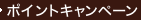•### Numerical Computation of Internal and External Flows : Computational Methods for Inviscid and Viscous Flows (Wiley Series in Numerical Methods in Engi 〈002〉

• 提携先の海外書籍取次会社に在庫がございます。通常2週間で発送いたします。
重要ご説明事項
1. 納期遅延や、ご入手不能となる場合が若干ございます。
2. 複数冊ご注文の場合、分割発送となる場合がございます。
3. 美品のご指定は承りかねます。
• ≪洋書のご注文につきまして≫ 「海外取次在庫あり」および「国内仕入れ先からお取り寄せいたします」表示の商品でも、納期の目安期間内にお届けできないことがございます。あらかじめご了承ください。

• 製本 Paperback:紙装版/ペーパーバック版
• 言語 ENG
• 商品コード 9780471924524
• DDC分類 620.1064

### Full Description

Numerical Computation of Internal and External Flows Volume 2Computational Methods for Inviscid and Viscous Flows C. Hirsch, Vrije Universiteit Brussel, Brussels, Belgium This second volume deals with the applications of computational methods to the problems of fluid dynamics. It complements the first volume to provide an excellent reference source in this vital and fast growing area. The author includes material on the numerical computation of potential flows and on the most up-to-date methods for Euler and Navier-Stokes equations. The coverage is comprehensive and includes detailed discussion of numerical techniques and algorithms, including implementation topics such as boundary conditions. Problems are given at the end of each chapter and there are comprehensive reference lists. Of increasing interest, the subject has powerful implications in such crucial fields as aeronautics and industrial fluid dynamics. Striking a balance between theory and application, the combined volumes will be useful for an increasing number of courses, as well as to practitioners and researchers in computational fluid dynamics.Contents Preface Nomenclature Part V: The Numerical Computation of Potential Flows Chapter 13 The Mathematical Formulations of the Potential Flow Model Chapter 14 The Discretization of the Subsonic Potential Equation Chapter 15 The Computation of Stationary Transonic Potential Flows Part VI: The Numerical Solution of the System of Euler Equations Chapter 16 The Mathematical Formulation of the System of Euler Equations Chapter 17 The Lax - Wendroff Family of Space-centred Schemes Chapter 18 The Central Schemes with Independent Time Integration Chapter 19 The Treatment of Boundary Conditions Chapter 20 Upwind Schemes for the Euler Equations Chapter 21 Second-order Upwind and High-resolution Schemes Part VII: The Numerical Solution of the Navier-Stokes Equations Chapter 22 The Properties of the System of Navier-Stokes Equations Chapter 23 Discretization Methods for the Navier-Stokes Equations Index

### Table of Contents

`THE NUMERICAL COMPUTATION OF POTENTIAL FLOWS.  The Mathematical Formulations of the  Potential Flow Model.  The Discretization of the Subsonic Potential  Equation.  The Computation of Stationary Transonic  Potential Flows.THE NUMERICAL SOLUTION OF THE SYSTEM OF EULEREQUATIONS.  The Mathematical Formulation of the System of  Euler Equations.  The Lax-Wendroff Family of Space-Centered  Schemes.  The Central Schemes with Independent Time  Integration.  The Treatment of Boundary Conditions.  Upwind Schemes for the Euler Equations.  Second Order Upwind and High-Resolution  Schemes.THE NUMERICAL SOLUTION OF THE NAVIER-STOKESEQUATIONS.  The Properties of the System of Navier-Stokes  Equations.  Discretization Methods for the Navier-Stokes  Equations.  Index.`